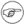# 8.1 运算符

LinuxStoryLinuxBash大约 6 分钟

# # 8.1 运算符

## # 赋值运算符

### # =

``````var=27
category=minerals  # "="左右不允许有空格
``````注意，不要混淆`=`赋值运算符与`=`测试操作符open in new window

``````#   =   作为测试操作符

if [ "\$string1" = "\$string2" ]
then
command
fi

#  [ "X\$string1" = "X\$string2" ] 这样写是安全的,
#  这样写可以避免任意一个变量为空时的报错。
#  (变量前加的"X"字符规避了变量为空的情况)
``````

## # 算术运算符

### # **

``````# Bash, 2.02版本，推出了"**"幂运算操作符。

let "z=5**3"    # 5 * 5 * 5
echo "z = \$z"   # z = 125
``````

### # %

``````bash\$ expr 5 % 3
2
``````

5/3=1，余2 取余运算符经常被用于生成一定范围内的数( 案例9-11, 案例9-15)，以及格式化程序输出(案例 27-16，案例 A-6)。 取余运算符还可以用来产生素数（案例A-15），取余的出现大大扩展了整数的算术运算。

``````#!/bin/bash
# gcd.sh: 最大公约数
#         使用欧几里得算法

#  两个整数的最大公约数（gcd）
#  是两数能同时整除的最大数

#  欧几里得算法使用辗转相除法
#    In each pass,
#       dividend <---  divisor
#       divisor  <---  remainder
#    until remainder = 0.
#    The gcd = dividend, on the final pass.
#
#  关于欧几里得算法更详细的讨论，可以查看:
#  Jim Loy's site, http://www.jimloy.com/number/euclids.htm.

# ------------------------------------------------------
# 参数检查
ARGS=2

if [ \$# -ne "\$ARGS" ]
then
echo "Usage: `basename \$0` first-number second-number"
fi
# ------------------------------------------------------

gcd ()
{

dividend=\$1             #  随意赋值，
divisor=\$2              #  两数谁大谁小是无关紧要的，
#  为什么?

remainder=1             #  如果在测试括号里使用了一个未初始化的变量，
#  会报错的。

until [ "\$remainder" -eq 0 ]
do    #  ^^^^^^^^^^  该变量必须在使用前初始化！
let "remainder = \$dividend % \$divisor"
dividend=\$divisor     # 对被除数，除数重新赋值
divisor=\$remainder
done                    # 欧几里得算法

}                         # 最后的 \$dividend 就是最大公约数（gcd）

gcd \$1 \$2

echo; echo "GCD of \$1 and \$2 = \$dividend"; echo

# 练习 :
# ---------
# 1) 检查命令行参数，保证其为整数，
#+   如果有错误，捕捉错误并在脚本退出前打印出适当的错误信息。
# 2) 使用本地变量(local variables)重写gcd()函数。

exit 0
``````

### # 小结

``````#!/bin/bash
# 使变量自增1，10种不同的方法实现

n=1; echo -n "\$n "

let "n = \$n + 1"   # 可以使用 let "n = n + 1"
echo -n "\$n "

: \$((n = \$n + 1))
#  ":" 是必要的，不加的话，bash会将
#+ "\$((n = \$n + 1))"看做一条命令。
echo -n "\$n "

(( n = n + 1 ))
#  更简洁的写法。
#  感谢 David Lombard指出。
echo -n "\$n "

n=\$((\$n + 1))
echo -n "\$n "

: \$[ n = \$n + 1 ]
#  ":" 是必要的，不加的话，bash会将
#+ "\$[ n = \$n + 1 ]"看做一条命令。
#  即使"n"是字符串，也是可行的。
echo -n "\$n "

n=\$[ \$n + 1 ]
#  即使"n"是字符串，也是可行的。
#* 不要用这种写法，它已被废弃且不具有兼容性。
#  感谢 Stephane Chazelas.
echo -n "\$n "

# 使用C风格的自增运算符也是可以的
# 感谢 Frank Wang 指出。

let "n++"          # let "++n" 可行
echo -n "\$n "

(( n++ ))          # (( ++n ))  可行
echo -n "\$n "

: \$(( n++ ))       # : \$(( ++n )) 可行
echo -n "\$n "

: \$[ n++ ]         # : \$[ ++n ] 可行
echo -n "\$n "

echo

exit 0
``````

``````echo \$BASH_VERSION   # Bash 1.14版本

a=2147483646
echo "a = \$a"        # a = 2147483646
let "a+=1"           # 自增 "a".
echo "a = \$a"        # a = 2147483647
let "a+=1"           # 再次自增"a"，超出取值范围。
echo "a = \$a"        # a = -2147483648
#      错误：超出范围，
#+     最左边的符号位被重置，
#+     结果变负
``````

Bash版本 >= 2.05b, Bash支持了64-bit整型数。注意，Bash并不支持浮点运算，Bash会将带小数点的数看做字符串。

``````a=1.5

let "b = \$a + 1.3"  # 报错
# t2.sh: let: b = 1.5 + 1.3: syntax error in expression
#                            (error token is ".5 + 1.3")

echo "b = \$b"       # b=1
``````

## # 位运算

### # <<=

`let "var <<= 2"` 的结果是var变量的值向左移了2位(乘以4)

## # 逻辑(布尔)运算符

### # !

``````if [ ! -f \$FILENAME ]
then
...
``````

### # &&

``````if [ \$condition1 ] && [ \$condition2 ]
#  等同于:  if [ \$condition1 -a \$condition2 ]
#  返回true如果 condition1 和 condition2 同时为真...

if [[ \$condition1 && \$condition2 ]]    # 可行
#  注意，&& 运算符不能用在[ ... ]结构里。
``````&&也可以被用在`list`结构中连接命令。

### # ||

``````if [ \$condition1 ] || [ \$condition2 ]

#  等同于:  if [ \$condition1 -a \$condition2 ]
#  返回true如果 condition1 和 condition2 任意一个为真...

if [[ \$condition1 || \$condition2 ]]    # 可行
#  注意，|| 运算符不能用在[ ... ]结构里。
``````

### # 小结

``````#!/bin/bash

a=24
b=47

if [ "\$a" -eq 24 ] && [ "\$b" -eq 47 ]
then
echo "Test #1 succeeds."
else
echo "Test #1 fails."
fi

#  错误:   if [ "\$a" -eq 24 && "\$b" -eq 47 ]
#          这样写的话，bash会先执行'[ "\$a" -eq 24'
#          然后就找不到右括号']'了...
#
#  注意:  if [[ \$a -eq 24 && \$b -eq 24 ]]  这样写是可以的
#  双方括号测试结构比单方括号更加灵活。
#  (双方括号中的"&&"与单方括号中的"&&"意义不同)
#  感谢 Stephane Chazelas 指出。

if [ "\$a" -eq 98 ] || [ "\$b" -eq 47 ]
then
echo "Test #2 succeeds."
else
echo "Test #2 fails."
fi

#  使用 -a 和 -o 选项也具有同样的效果。
#  感谢 Patrick Callahan 指出。

if [ "\$a" -eq 24 -a "\$b" -eq 47 ]
then
echo "Test #3 succeeds."
else
echo "Test #3 fails."
fi

if [ "\$a" -eq 98 -o "\$b" -eq 47 ]
then
echo "Test #4 succeeds."
else
echo "Test #4 fails."
fi

a=rhino
b=crocodile
if [ "\$a" = rhino ] && [ "\$b" = crocodile ]
then
echo "Test #5 succeeds."
else
echo "Test #5 fails."
fi

exit 0
``````

`&&``||`运算符也可以用在算术运算中。

``````bash\$ echo \$(( 1 && 2 )) \$((3 && 0)) \$((4 || 0)) \$((0 || 0))
1 0 1 0
``````

## # 其他运算符

### # ,

``````let "t1 = ((5 + 3, 7 - 1, 15 - 4))"
echo "t1 = \$t1"           ^^^^^^  # t1 = 11
# 这里的t1 被赋值了11，为什么？

let "t2 = ((a = 9, 15 / 3))"      # 对"a"赋值并对"t2"求值。
echo "t2 = \$t2    a = \$a"         # t2 = 5    a = 9
``````

[^1]: 取决与不同的上下文，+= 也可能作为字符串连接符。它可以很方便地修改环境变量。 [^2]: 副作用，顾名思义，就是预料之外的结果。